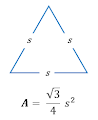## C program to find area of an equilateral triangle

Write a C program to input side of an equilateral triangle from user and find area of the given triangle. How to find area of an equilateral triangle in C programming. C program to calculate area of an equilateral triangle if its side is given.

#### Required knowledge

Operators, Data Types in c, Variables in C, Basic input/output

#### Area of an equilateral triangle

Area of an equilateral triangle is given by formula.C equivalent expression to find area of equilateral triangle - (sqrt(3) / 4) * (side * side)

#### Logic to find area of equilateral triangle

Mathematical formula for area of equilateral triangle in programming notation can be written as (sqrt(3) / 4) * (side * side). Where sqrt() is a function used to compute square root. We will use this formula to find area of equilateral triangle.

Read more - Program to find square root of a number

Below is the step by step descriptive logic to find area of an equilateral triangle.

1. Input side of the equilateral triangle. Store it in some variable say side.

2. Use the formula of equilateral triangle to find area i.e. area = (sqrt(3) / 4) * (side * side).

3. Finally, print the value of resultant area.

#### Program to find area of an equilateral triangle

```

/**
* C program to find area of an equilateral triangle
*/

#include <stdio.h>
#include <math.h> // Used for sqrt() function

int main()
{
float side, area;

/* Input side of equilateral triangle */
printf("Enter side of an equilateral triangle: ");
scanf("%f", &side);

/* Calculate area of equilateral triangle */
area = (sqrt(3) / 4) * (side * side);

/* Print resultant area */
printf("Area of equilateral triangle = %.2f sq. units", area);

return 0;
}
```

sqrt() function is used to calculate square root of a number.

##### Output:
```
Enter side of an equilateral triangle: 75
Area of equilateral triangle = 2435.69 sq. units
```
```
Recommended posts

Loop programming exercises index.
Basic programming exercises index.
If else programming exercises index.
Array programming exercises index.
String programming exercises index.
Pointer programming exercises index.
Star Patterns exercises index.
Number Patterns exercises index.
alphabet Patterns exercises index.
series program exercises index.

```